# CBSE Class 10 Science Magnetic Effects Of Current Worksheet Set B

Read and download free pdf of CBSE Class 10 Science Magnetic Effects Of Current Worksheet Set B. Students and teachers of Class 10 Science can get free printable Worksheets for Class 10 Science Chapter 13 Magnetic Effect of Electric Current in PDF format prepared as per the latest syllabus and examination pattern in your schools. Class 10 students should practice questions and answers given here for Science in Class 10 which will help them to improve your knowledge of all important chapters and its topics. Students should also download free pdf of Class 10 Science Worksheets prepared by school teachers as per the latest NCERT, CBSE, KVS books and syllabus issued this academic year and solve important problems with solutions on daily basis to get more score in school exams and tests

## Worksheet for Class 10 Science Chapter 13 Magnetic Effect of Electric Current

Class 10 Science students should refer to the following printable worksheet in Pdf for Chapter 13 Magnetic Effect of Electric Current in Class 10. This test paper with questions and answers for Class 10 will be very useful for exams and help you to score good marks

### Class 10 Science Worksheet for Chapter 13 Magnetic Effect of Electric Current

Question. A rectangular loop carrying a current i is situated near a long straight wire such that the wire is parallel to one of the sides of the loop and is in the plane of the loop. If a steady current i is created in wire as shown in figure below, then the loop will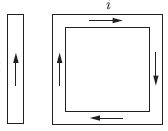(a) rotate about an axis parallel to the wire
(b) move towards the wire
(c) move away from the wire or towards right
(d) remain stationary

26. A copper wire is held between the poles of a magnet.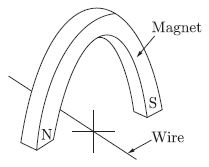The current in the wire can be reversed. The pole of the magnet can also be changed over. In how many of the four directions shown can the force act on the wire?
(a) 1
(b) 2
(c) 3
(d) 4

Question. The magnetic lines of force, inside a current carrying solenoid, are
(a) along the axis and are parallel to each other
(b) perpendicular to the axis and equidistance from each other
(c) circular and they do not intersect each other
(d) circular at the ends but they are parallel to the axis inside the solenoid.

27. A circular loop placed in a plane perpendicular to the plane of paper carries a current when the key is ON. The current as seen from points A and B (in the plane of paper and on the axis of the coil) is anti clockwise and clockwise respectively. The magnetic field lines point from B to A. The N-pole of the resultant magnet is on the face close to
(a) A
(b) B
(c) A if the current is small, and B if the current is large

(d) B if the current is small and A if the current is large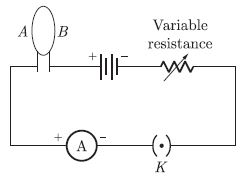Question. Instrument can be shielded from outside magnetic effects by surrounding them with
(a) Rubber shield
(b) Glass shield
(c) Iron shield
(d) Brass shield

Question. Four metal rods are placed, in turn, inside a coil of copper wire.The table below gives the results of the experiment.
Which rod would be the most suitable to use for the case of a coil in a circuit breaker?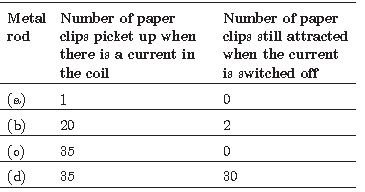Question. The power of an electric motor can be increased by
a. By decreasing the area of cross – section of the coil.
b. By increasing the number of turns in the coil.
c. All of these
d. By decreasing the strength of the magnetic field.
Explanation: The power of an electric motor can be increase by one or all of the following means:
i. By increasing the number of turns in the coil.
ii. By increasing the area of cross section of the coil.
iii. By increasing the strength of the radical magnetic field.
iv. By increasing the magnitude of the current flowing the coil.

Question. Two organs where magnetic field is produced are
(a) Heart and lungs
(b) Heart and brain
(c) Brain and lungs
(d) Heart and Liver

Question. In the statement of Fleming’s left hand rule. What does the fore finger represent?
a. electric field
b. force
c. current
d. magnetic field
Explanation: The direction of force which acts on the current-carrying
conductor placed in a magnetic field is given by Fleming’s left hand rule. It states that if the forefinger, thumb and middle finger of left hand are stretched mutually perpendicular and the forefinger points along the direction of external magnetic field, middle finger indicates the direction of current, then thumb points along the direction of force acting on the conductor.

Question. A commutator changes the direction of current in the coil of
a. a DC motor and an AC generator
b. an AC generator
c. a DC motor and a DC generator
d. a DC motor
Explanation: The commutation in DC machine or more specifically commutation in DC generator is the process in which generated alternating current in the armature winding of a dc machine is converted into direct current after going through the commutator and the stationary brushes. Again in DC Motor, the input DC is to be converted in alternating form in armature and that is also done through commutation. This transformation of current from the rotating armature of a DC machine to the stationary brushes needs to maintain continuously moving contact between the commutator segments and the brushes.

Question. If the key in the arrangement is taken out (the circuit is made open) and magnetic field lines are drawn over the horizontal plane ABCD, the lines are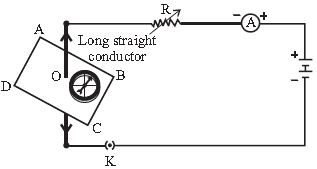(a) concentric circles
(b) elliptical in shape
(c) straight lines parallel to each other
(d) concentric circles near the point O but of elliptical shapes as we go away from it

Question. The rectangular coil of copper wires is rotated in a magnetic field. The direction of induced current change once in each
a. one fourth revolution
b. half revolution
c. one revolution
d. two revolutions
Explanation: When a rectangular coil of copper is rotated in a magnetic field, the direction of the induced current in the coil changes once in each half revolution as a result of which the direction of the current in the coil remains same.

Question. Transformer works on the principle of
a. Electro magnetic Induction
b. Electric field
c. Electricity
d. Magnetic field
Explanation: Transformer works on the principle of mutual induction of two coils or Faraday Law’s Of Electromagnetic induction. When current in the primary coil is changed the flux linked to the secondary coil also changes. Consequently an EMF is induced in the secondary coil due to Faraday law’s of electromagnetic induction.

Question. Choose the incorrect statement from the following regarding magnetic lines of force
(a) The direction of magnetic field at a point is taken to be the direction in which the north pole of a magnetic compass needle will point
(b) Magnetic field lines are closed curves
(c) If magnetic field lines are parallel and equidistant, they represent zero field strength
(d) Relative strength of magnetic field is shown by the degree of closeness of the field lines

Question. A circular loop placed in a plane perpendicular to the plane of paper carries a current when the key is ON.
The current as seen from points A and B (in the plane of paper and on the axis of the coil) is anti clockwise and clockwise respectively. The magnetic field lines point from B to A. The N-pole of the resultant magnet is on the face close to
(a) A
(b) B
(c) A if the current is small, and B if the current is large
(d) B if the current is small and A if the current is large

### A ssertion-Reason Type Questions

For question numbers 1 and 2, two statements are given-one labeled as Assertion (A) and the other labeled
Reason (R). Select the correct answer to these questions from the codes (a), (b), (c) and (d) as given below:
(a) Both ‘A’ and ‘R’ are true and ‘R’ is correct explanation of the Assertion.
(b) Both ‘A’ and ‘R’ are true but ‘R’ is not correct explanation of the Assertion.
(c) ‘A’ is true but ‘R’ is false.
(d) ‘A’ is false but ‘R’ is true.

Question. Assertion: Magnetic field lines do not intersect.
Reason: Magnetic field lines are closed curves.

Question. Assertion: A compass needle gets defected when electric current is passed through the nearly metallic wire.
Reason: The S.I unit of magnetic field strength is Oersted.

### Fill in The Blank

Question. Crowding the wires of a solenoid more closely together will .......... the strength of the field inside it.

Question. The S.I. unit of magnetic flux .........

Question. No force acts on a current carrying conductor when it is .......... to the magnetic field.

Question. The field lines about the wire consist of a series of concentric circles whose direction is given by the .......... rule.

Question. Magnetic fields are produced by ..........

Question. An electromagnet is a .......... magnet.

Question. An electric current can be used for making temporary magnets known as .........

Question. In an AC generator, maximum number of lines of force pass through the coil when the angle between the plane of coil and lines of force is ..........

Question. In any generator, the current in the armature is of the ...........type.

Question. The unit of magnetic field is ..........

Question. The frequency for A.C. (alternating current) in USA is ..........

Question. A compass needle is a .......... magnet.

Question. You are looking down the axis of a solenoid, and the current from your position is clockwise. The end of the solenoid facing you is a ......... pole.

Question. The armature in a motor rotates within a ......... field.

Question. The magnetic lines of force are the lines drawn in a magnetic field along which a ......... pole would move.

### Case Study Questions

Study this table related to wattage of home appliances and answer the questions that follow.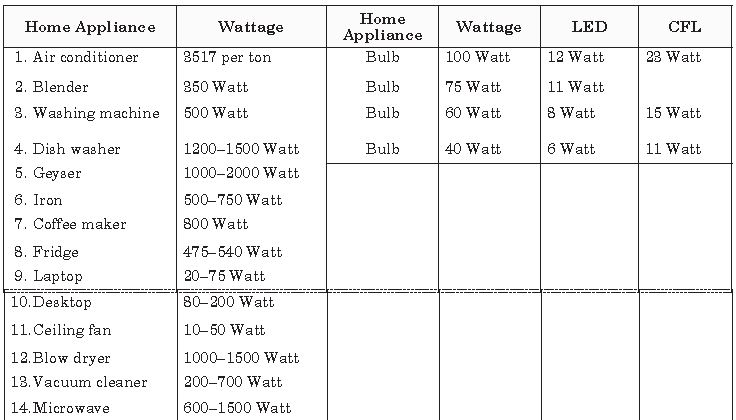Question. Which of the following home applicance is not based on magnetic effect of current?
(a) Ceiling fan
(b) Blender
(c) Geyser
(d) Washing Machine

Question. Which of the following will to consume least power?
(a) Laptop
(b) Desktop
(c) Printer
(d) Blender

Question. Many appliance works on the principle of electromagnetic induction. The role which depicts the direction of induced current is as
(b) Henery’s rule
(c) Fleming’s rule
(d) Maxwell’s rule

Question. Which of the following will consume maximum power?
(a) Fridge
(b) Iron
(c) Air conditioner
(d) Coffee maker

Question. Which of the following lighting device is best to use in terms of power consumption?
(a) Bulb
(b) CFL
(c) Tube light
(d) LED

Question. The diagram given below represents the magnetic field caused by a current carrying conductor. Identify the nature of the coil.Answer : The nature of the coil is circular in form of loop.

Question. Why don’t two magnetic lines of force intersect each other?
Answer : No two magnetic field lines intersect each other because if they did, it would mean that at the point of intersection, the compass needle would point towards two direction, which is not possible

Question. How can it be proved that a magnetic field exists around a current carrying metallic wire?
Answer :  When a magnetic compass needle is placed close to the current carrying wire, it will get deflected.

Question. A bar magnet is placed between two iron bars. Draw a diagram to show the induced poles.
Answer : Both the iron bars get magnetised as shown in the figur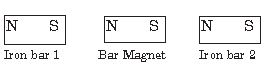Question. What type of field is around stationary charge ?
Answer :  A stationary charge cannot produce a magnetic field. It produces an electric field. Static charges are the charges at rest.

Question. An electric oven of 2kW power rating is operated in a domestic electric circuit (220 V) that has a current rating of 5A. What result do you expect ? Explain.
10. Power P = 2kW = 2,000 W, V = 220 volts, I = ?
P = VI or I =P/V
I = 2000/220  = 100/11 =9.09 A
A current of 9.09 A will flow in the circuit. Since the current rating of circuit is 5A, the fuse (of 5A) rating if inserted in circuit will burn up. If no fuse has been put in the circuit, there may be a fire.

Question. Draw a diagram to represent the uniform magnetic field in the region around the magnet.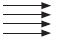Question. Mention the special feature regarding the shape of magnetic field lines.
Answer : Magnetic field lines are closed curves

Question. Mention the shape of the magnetic field lines around a current carrying straight conductor.
Answer :  The shape of magnetic field lines around the straight current carrying conductor is in the form of concentric circles.

Question. Identify the poles of the magnet as shown in the given figure:Answer : A1 represents North pole and B1 represents South pole.

Question. Why does a compass needle get deflected when brought near a bar magnet?
Answer : A compass needle behaves like a small bar magnet when it is brought near a bar magnet. Its magnetic field lines interact with that of bar magnet. Hence compass needle gets deflected.

Question. A current through a horizontal power line flows from east to west direction. What is the direction of magnetic field at a point directly below it and at a point directly above it?
Answer : At a point below it, the direction is from North to South and at a point above it, the direction is from South to North

Question. State the direction of magnetic field inside a bar magnet.
Answer : It is from South pole to North pole.

Question. If magnetic field lines are crossed at a point, what does it indicate?
OR
Explain why two magnetic lines of force do not intersect.
Answer : The magnetic lines of force do not intersect with each another due to the fact that resultant force on the north pole at any point can only be in one direction. But if the two magnetic lines of force intersect one another, then the resultant force on the north pole placed at the point of intersection will be along two directions, which is not possible

Question. If in a straight wire ‘A’, current is flowing in vertically downward direction whereas in the straight wire ‘B’ current is flowing in vertically upward direction. What is the direction of magnetic field (a) in wire ‘A’, (b) in wire ‘B’.?
Answer : (a) Anticlockwise, (b) Clockwise

Question. What type of core is used in electromagnets?

Question. When does an electric short circuit occurs?
Answer :  If either the insulation of wires used in an electric circuit is damaged or there is a fault in the appliances, live wire and neutral wire may come in direct contact. As a result, the current in the circuit abruptly rises and short circuiting occurs.

Question. The given figure shows a DC motor model used by a student to study electromagnetism. The two ends of the coil are fixed to a pair of curved elastic metal strips. The metal strips are connected to the power supply with a rheostat.
i. State the direction of rotation of the coil when viewed from the front.
ii. The student is still testing on the feasibility of using the metal strips in the model.
What is he trying to achieve?
Answer :  i. The direction of rotation of the coil is anti-clockwise when viewed from the front.
ii. He is trying to achieve the rotation of the coil in one direction. As the current in the coil reverses for every half turn, the coil rotates in one direction.

Question.  Why do not two magnetic field lines intersect each othe?
Answer : The two magnetic field lines can never intersect each other because two tangents can be drawn from that point of intersection which will give two directions of the field from the same point, which is impossible.

Question. An alternating electric current has a frequency of 50 Hz. How many times does it change its direction in one second? Give reason for your answer.
Answer : 100 times in one second because one complete cycle involves two times change of direction.

Question. Identify the type of magnetic fields represented by the magnetic field fines given below and name the type conductors which can produce them.Answer : a. Magnetic field lines of a current carrying circular loop.
b. Magnetic field lines in a solenoid.

Question. Define a solenoid. How is it different from a coil?
Answer : A coil of many circular turns of insulated wire wrapped closely in the form of a cylinder is called a solenoid.
In a current carrying solenoid magnetic field is same at all points inside it, i.e. field is uniform inside the solenoid. The magnetic field inside a coil vary.

Question. On what factors does the force experienced by a current carrying conductor placed in a uniform magnetic field depend?
Answer : The force experienced by a current carrying conductor in a magnetic field depends upon—
a. length of the conductor
b. current in the conductor
c. magnetic field in which it is kept

Question. Name any three factors on which the magnitude of the magnetic field due to solenoid depends.
Answer : Factors on which the magnitude of the magnetic field due to solenoids
a. number of turns in the solenoid
b. area of cross section of the coil
c. strength of current in solenoid

Question. State the condition for electromagnetic induction to take place. A cylindrical bar magnet is kept along the axis of a circular coil as shown in the figure. Will there be a current induced in the coil if the magnet is rotated about its axis? Discuss.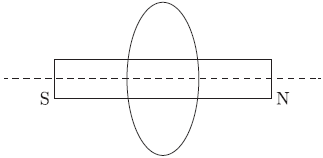a. Either coil or magnet or both must have a relative motion between them.
b. If there is a change in current in a coil then current will be induced in the another neighbouring coil. No current is induced because there is no change in magnetic field.

Question. Write one difference between direct current and alternating current. Which one of the two is mostly produced at power stations in our country? Name one device which provides alternating current. State one important advantage of using alternating current.
Answer : Direct current has constant magnitude and unidirectional current. Alternating current changes its magnitude and direction alternatively.
a. Alternating current is produced in power stations in India.
b. AG generators are used to produce AC.
c. AC voltage can be increased or decrease with the help of a transformers.

Question. An alpha particle (+ve charged particle) enters a magnetic field at right angle to it as shown in figure.
Explain with the help of a relevant rule, the direction of force acting on the alpha particle.Answer : Force on a-particle will be in the upward direction as per Fleming’s left hand rule.

Question. What is the nature of magnetic field produced by a straight current carrying circular coil? Explain with the help of an experiment.
Answer : Bend a wire in the shape of a circular loop. Pass the coil through a cardboard. Connect the free ends of the coil to a battery and a key. Sprinkle some iron filings on the cardboard. Put on the key you will find that the iron filings arrange themselves in the form of concentric circles. The magnetic lines of forces near each segment of wire are circular and form concentric circles, whereas the lines of force near the centre of the coil are almost straight lines.
The right hand thumb rule predicts the direction of this magnetic field it states “Grasp the conductor in the right hand with the thumb pointing in the direction of current, and then the direction in which the fingers curl gives the direction of the magnetic field.”

Question. State one main difference between ac and dc. Why ac is preferred over dc for long range transmission of electric power? Name one source each of dc and ac.
Answer : The magnitude and direction of ac remains same whereas a.c. changes its magnitude and direction periodically. Low AC voltage can be increase to high voltage to prevent loss in electric energy during its long distance transmission.
AC generator and DC generator/or cell.

Question. What is meant by the term “magnetic field lines”?
List two properties of magnetic field lines.
Answer : Magnetic field lines are the path traced by a hypothetical north pole in the magnetic field of another magnet or current carrying conductor if it is allowed to do so.
Properties:
a. No two field lines are found to cross each other.
b. Outside the magnet magnetic field lines travel from N-pole to S-pole and inside the magnet from S-pole to N-pole.

Answer : When live wire and neutral wire come in contact with each other short circuit takes place. This may be due to fault in electric circuit or when insulation of wire is damaged. During the short circuiting current increases abruptly due to decrease in resistance in the circuit. This short circuiting leads the spark and house may catch fire.
Over loading means to draw the current by all electric appliances in the circuit more than maximum permitted value of the current, overloading also leads the heating of wires which may damage the insulation of wires.

Question. (a) A compass needle gets deflected when brought near a current carrying conductor. Why?
(b) What happened to the reflection of needle when current in the conductor is increased?
Answer : (a) Near a current carrying conductor a magnetic field is produced which deflect the compass.
(b) With the increase in strength of current in the conductor strength of magnetic field also increases so there will be more deflection in compass.

Question. Draw the patterns of magnetic field fines due to a bar magnet. The magnetic field fines are closed curves. Why?
Answer : Magnetic field lines emerge out from the N-pole of a bar magnet go to the S-pole and inside the magnet field lines goes from S-pole to N-pole thus form the closed curves.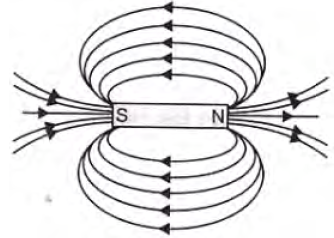Question. State the characteristics of magnetic force.
Answer : If a charge is moving in a magnetic field then it experiences a force known as magnetic force F = qVBsinθ , B is magnetic force, θ is angle between B and V. This magnetic force
a. acts only when charge is moving
b. does not act when charge is at rest
c. is maximum when charge is moving perpendicular to the magnetic field
d. does not act when charge is moving in the same direction of magnetic field.

Question. What are permanent magnet and electromagnet?
Give two uses of each.
Answer : Permanent Magnet: It has constant magnetic field around it. e.g. generator, loud speaker. Electromagnet: When a soft iron is placed in a solenoid it gets magnetised till there is current in solenoid, e.g. electric bells, cranes.

Question. With the help of a neat diagram, describe how you can generate induced current in a circuit.
Answer : Take a coil of large number of turns and connect the two ends of the coil with a galvanometer. Galvanometer is a device used to detect the presence of current.
Now bring N pole of a strong magnet towards the coil. There is a momentary deflection in the galvanometer. Now bring the N pole away from the coil, again there is a deflection in the opposite direction in the galvanometer deflection in the galvanometer shows that a current is induced in the coil. The induced current can be produced by keeping magnet stationary and giving movement to the coil, or when both have a relative motion w.r.t. each other.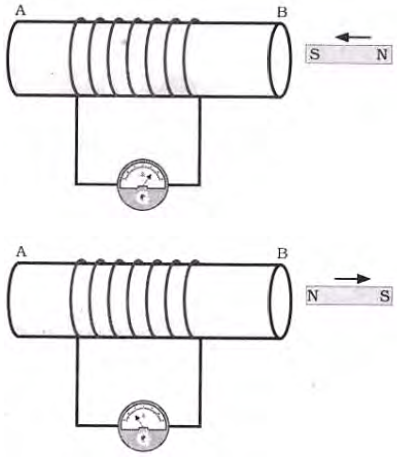Question. Why is series arrangement not used for domestic circuits?
Answer : In domestic circuits, all appliances are connected in parallel not in series because
a. In series combination same current is passed through each appliances whereas they may need different current.
b. If one appliance fails to work then circuit will be broken and all other appliances will stop to work.
c. If one appliance is switched off others also will stop working i.e. all appliance will work together whether we require it or not.
d. In series combination total potential difference is divided among all appliances in proportion to their resistance. So all appliance will not get required voltage to operate efficiently.

Question. Explain briefly different methods of producing induced emf.
Answer : a. By mutual induction: If we change current in one coil, a current is induced in the neighbouring coil.
b. By giving a relative motion to a magnet and coil in its neighbourhood.

Question. What is the role of fuse, used in series with any electrical appliance? Why should a fuse with defined rating not be replaced by one with a larger rating?
Answer : Fuse wire is safety device to prevent electrical devices due to short circuiting or overloading. The fuse wire is rated for a maximum current which has high resistance and low melting point. When there is short circuiting large current is passed in the circuit. Due to large current in fuse wire heat is produced and by melting fuse wire breaks the circuit to keep other appliances safe.
If a fuse wire is replaced by an ordinary copper wire which has low resistance and high melting point it will not melt and domestic appliance may get damaged due to excessive heat due to short circuiting or overloading.

Question. Magnetic field lines are shown in the given diagram. A student makes a statement that magnetic field at X is stronger than at Y. Justify this statement. Also, redraw the diagram and mark the direction of magnetic field lines.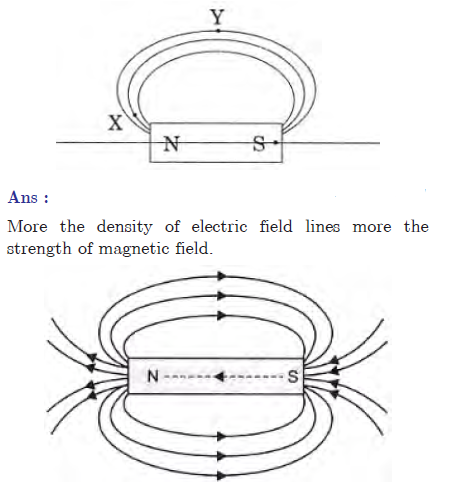Question. What is meant by the term magnetic field lines? List any two properties of magnetic field lines.
Answer : The imaginary path traced by N-pole of a magnet in a magnetic field is called magnetic field lines. Properties:
a. Two magnetic field lines never intersect each other.
b. Magnetic field lines are crowded near the poles and near the mid part of magnets the density of magnetic lines of force decreases.

Question. Draw a diagram to show how a magnetic needle deflects when it is placed above or below a straight conductor carrying current depending on the direction of the current in the conductor.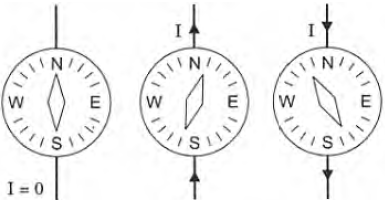Explain two possible causes due to which overloading may occur in household circuit. Explain one precaution that should be taken to avoid the overloading of domestic electric circuit.
Answer : Connecting large number of electric appliances in one socket whose load is more than the maximum permitted limit. The two possible cause are
a. all of a sudden supply of high voltage and
b. too many devices connected in a single socket.
Precautions:
a. use of voltage regulator,
b. load of a socket must be greater than its permitted rating.

Question. Name and state the rule to determine the direction of a force experienced by a current carrying straight conductor placed in a magnetic field which is perpendicular to it. Name a device that uses current carrying conductor and magnetic field.
Answer : Fleming’s left hand rule gives the direction of force experienced by a current carrying straight conductor placed in a magnetic field which is perpendicular to it. According to Fleming’s left hand rule if we stretch our left hand thumb, forefinger and middle finger in such a way that forefinger points the direction of magnetic field, middle finger points the direction of current then thumb will give the direction of force on the conductor.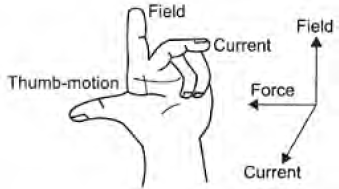Question. (a) State Fleming’s left hand rule.
(b) Write the principle of working of an electric motor.
(c) Explain the function of the following parts of an electric motor.
(i) Armature, (ii) Brushes, (iii) Split ring
Answer : (a) Fleming’s left hand rule: If we stretch thumb, forefinger and middle finger of left hand perpendicular to each other in such a way that forefinger points in the direction of magnetic field, middle finger points in the direction of current then the thumb will point in the direction of motion/force on the current carrying conductor.
(b) Principle of working of electric motor: A current carrying coil placed in a magnetic field experience a torque. If the coil is free to move it will rotate in the magnetic field.
(c) (i) Armature: The soft iron core on which the coil is wound along with coils is called armature which enhances the power of motor.
(ii) Brushes: Brushes help in transfer of current between coil and external circuit.
(iii) Split rings reverses the direction of current after every half rotation of the coil so that the direction of torque/force on the coil remains and it continuous to rotate.

Question. How does the strength of the magnetic field at the centre of a circular coil of a wire depend on:
b. number of turns in the coil.
Answer : Magnetic field strength at the centre of a circular coil of a wire carrying current
a. ∝ 1/r , i.e. magnetic field decreases with increase in radius.
b. ∝ n, i.e. magnetic field increases with increase in number of turns.

Question. A uniform magnetic field is directed vertically upwards. In which direction in this field should an a-particle (which are positively charged particles) be projected so that it is deflected south ward? Name and state the rule you have used to find the direction in this case.
Answer : Forefinger - direction of magnetic field (upwards). Thumb - direction of force (southwards) Middle finger gives direction of current or direction of positively charged particle (East) i.e. from west to east a particles must be projected.

Question. A magnetic compass shows a deflection when placed near a current carrying wire. How will the deflection of the compass get affected if the current in the wire is increased? Support your answer with a reason.
Answer : If current is increased magnetic field around this conductor increases, the deflection in the galvanometer also increases.

Question. No two magnetic field lines can intersect each other. Explain.
Answer : If two magnetic field lines intersect each other then at the point of intersection there will be two different tangents pointing two different directions of magnetic field at a point which is not possible.

Question. For the circular coil carrying current shown alongside, draw magnetic field lines. Decide which of its face behaves as North Pole and which face as South Pole.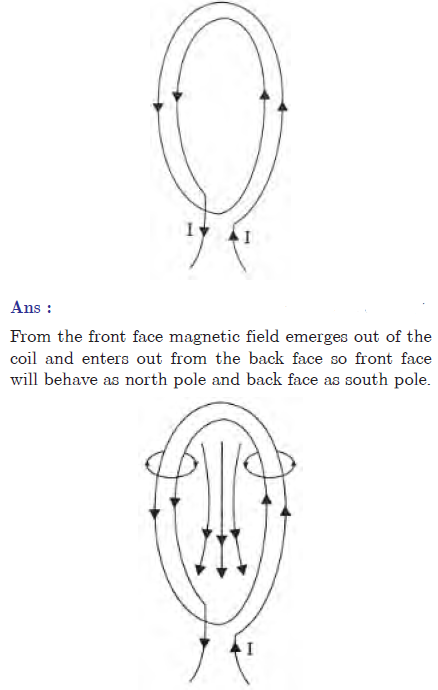Question. Why does a current carrying Conductor experiences a force when it is placed in a magnetic field? State Fleming’s left hand rule.
Answer : When a current carrying conductor is placed in a magnetic field it experiences a force due to interaction between the two magnetic fields due to current carrying , conductor and given magnetic field of a magnet. If we hold our thumb, forefinger, and middle finger perpendicular to each other in such a way that forefinger points the direction of magnetic field, middle finger points the direction of charge q (direction of current in conductor), then thumb will point the direction force acting upon the conductor.

Question. What are magnetic field lines? How is the direction of magnetic field at a point determined? Mention few important properties of magnetic field lines.
Answer : Magnetic field lines are the path traced by north pole in a magnetic field. A tangent drawn to the magnetic field lines gives the direction of magnetic field at that point.
a. Magnetic field lines emerge out from the N-pole and go to the south pole and from S-pole to N-pole inside the magnet forming the closed curve.
b. They do not intersect each other.

Question. (a) Name the type of electric current generated by the most of the power stations in our country.
(b) Why is it preferred over the other type?
(c) State the frequency of the power supply generated in India.
(b) AC can be transmitted at high voltage using step up transformer to prevent loss in transmission.
(c) 50 Hz

Question. (a) In a pattern of magnetic field fines due to a bar magnet, how can the regions of relative strength be identified?
(b) Compare the strength of field near the poles and middle of a bar magnet.
Answer : (a) More the density of the magnetic field lines i.e. more closer the magnetic field lines more the strength of magnetic field.
(b) Near the poles magnetic field lines are closer than the middle of a bar magnet. So magnetic strength is more near poles than middle part of bar magnet.

Question. Define electromagnetic induction? Two circular coils A and B are placed close to each other. If the current in the coil A is changed, will some current be induced in the coil B? Explain.
Answer :  Electromagnetic induction means the production of induced current in a closed coil due to the change in the magnetic field.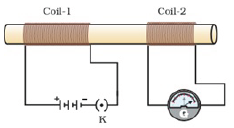When current flows in coil-1, magnetic flux sets up around coil A due to which some magnetic field sets up around coil-2 and when current in coil-1changes, magnetic field around coil-2 also changes thus some induced current flow through coil B due to which galvanometer deflects.

Question. Explain the underlying principle of working of an electric generator by drawing a labelled diagram. What is the function of brushes.
Answer : Principle: Alternators are based on the principle that an induced current is produced in a conductor whenever there is change in magnetic lines of force linked with it. The direction on induced current is given by Fleming's right hand rule stated below.
Fleming's right hand rule. If the thumb, forefinger and central finger of right hand are stretched out mutually at right angles to each other such that forefinger points along the direction of magnetic field and thumb along the direction of motion of the conductor, then the central finger indicates the direction of the current produced in the coil.
Construction: A simple A.C. generator consists of the following important parts :
Working : Let the coil initially be in horizontal position as shown in fig. Let the coil be rotated in anticlockwise direction between the pole pieces N and S of a horseshoe magnet.
At an instant, let AB move down and CD move up as shown in the fig. The coil AB cut the magnetic lines of force near N-pole of the magnet, side CD cut the lines near S pole of the magnet. Due to the change in the magnetic field cutting the coil, an induced current is produced in AB and DC. Using Fleming's right hand rule to side AB and DC of the coil, we observe that the current flows in the direction B and A and D and C. Effective current flows along DCBA.
After half rotation, side AB and DC of the coil will interchange their positions. AB will come towards right and DC towards left. Now AB starts moving up and DC downwards.
The direction of induced current in each side is reversed after half a revolution. Due to the change in the direction of induced current in the coil is reversed after half revolution, hence positive or negative polarity of the two ends is also changed.
In India, we produce 50 Hz A.C. Hence coil is to be rotated 50 times per second. In a single rotation, the current changes its direction twice i.e. each termed is positive for only  1/2 x 50 = 1/100 = 0.01s and negative for 0.01s. Since the current alternates its direction every 0.01 s. the current produced is called alternating current or A.C.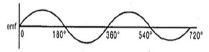i. Armature: It consists of coil ABCD having a large number of insulated copper wires wound over a soft iron core. The coil is called armature. The armature is mounted on an axle which can be rotated by force exerted by falling water, wind or stream.ii. Field magnets : The coil is held between the pole pieces of a strong magnet called the field magnet. In small dynamos, the magnetic field is provided by permanent magnet.
in large dynamos, the magnetic field is provided by electromagnets.
iii. Split Rings : These consist of two hollow metallic ring R1 and R2 mounted on the axle of the coil. The ends of the limbs AB and CD of the coil are connected to the rings R1 and R2 respectively. These rings rotate along with the rotation of The armature.
iv. Current from B1, B2 is taken out to load connected across two brushes.

## More Study Material

### CBSE Class 10 Science Chapter 13 Magnetic Effect of Electric Current Worksheet

We hope students liked the above worksheet for Chapter 13 Magnetic Effect of Electric Current designed as per the latest syllabus for Class 10 Science released by CBSE. Students of Class 10 should download in Pdf format and practice the questions and solutions given in the above worksheet for Class 10 Science on a daily basis. All the latest worksheets with answers have been developed for Science by referring to the most important and regularly asked topics that the students should learn and practice to get better scores in their class tests and examinations. Studiestoday is the best portal for Class 10 students to get all the latest study material free of cost.

### Worksheet for Science CBSE Class 10 Chapter 13 Magnetic Effect of Electric Current

Expert teachers of studiestoday have referred to the NCERT book for Class 10 Science to develop the Science Class 10 worksheet. If you download the practice worksheet for one chapter daily, you will get higher and better marks in Class 10 exams this year as you will have stronger concepts. Daily questions practice of Science worksheet and its study material will help students to have a stronger understanding of all concepts and also make them experts on all scoring topics. You can easily download and save all revision worksheet for Class 10 Science also from www.studiestoday.com without paying anything in Pdf format. After solving the questions given in the worksheet which have been developed as per the latest course books also refer to the NCERT solutions for Class 10 Science designed by our teachers

#### Chapter 13 Magnetic Effect of Electric Current worksheet Science CBSE Class 10

All worksheets given above for Class 10 Science have been made as per the latest syllabus and books issued for the current academic year. The students of Class 10 can be rest assured that the answers have been also provided by our teachers for all worksheet of Science so that you are able to solve the questions and then compare your answers with the solutions provided by us. We have also provided a lot of MCQ questions for Class 10 Science in the worksheet so that you can solve questions relating to all topics given in each chapter. All study material for Class 10 Science students have been given on studiestoday.

#### Chapter 13 Magnetic Effect of Electric Current CBSE Class 10 Science Worksheet

Regular worksheet practice helps to gain more practice in solving questions to obtain a more comprehensive understanding of Chapter 13 Magnetic Effect of Electric Current concepts. Worksheets play an important role in developing an understanding of Chapter 13 Magnetic Effect of Electric Current in CBSE Class 10. Students can download and save or print all the worksheets, printable assignments, and practice sheets of the above chapter in Class 10 Science in Pdf format from studiestoday. You can print or read them online on your computer or mobile or any other device. After solving these you should also refer to Class 10 Science MCQ Test for the same chapter.

#### Worksheet for CBSE Science Class 10 Chapter 13 Magnetic Effect of Electric Current

CBSE Class 10 Science best textbooks have been used for writing the problems given in the above worksheet. If you have tests coming up then you should revise all concepts relating to Chapter 13 Magnetic Effect of Electric Current and then take out a print of the above worksheet and attempt all problems. We have also provided a lot of other Worksheets for Class 10 Science which you can use to further make yourself better in Science

Where can I download latest CBSE Printable worksheets for Class 10 Science Chapter 13 Magnetic Effect of Electric Current

You can download the CBSE Printable worksheets for Class 10 Science Chapter 13 Magnetic Effect of Electric Current for latest session from StudiesToday.com

Can I download the Printable worksheets of Chapter 13 Magnetic Effect of Electric Current Class 10 Science in Pdf

Yes, you can click on the links above and download Printable worksheets in PDFs for Chapter 13 Magnetic Effect of Electric Current Class 10 for Science

Are the Class 10 Science Chapter 13 Magnetic Effect of Electric Current Printable worksheets available for the latest session

Yes, the Printable worksheets issued for Class 10 Science Chapter 13 Magnetic Effect of Electric Current have been made available here for latest academic session

How can I download the Class 10 Science Chapter 13 Magnetic Effect of Electric Current Printable worksheets

You can easily access the links above and download the Class 10 Printable worksheets Science Chapter 13 Magnetic Effect of Electric Current for each chapter

Is there any charge for the Printable worksheets for Class 10 Science Chapter 13 Magnetic Effect of Electric Current

There is no charge for the Printable worksheets for Class 10 CBSE Science Chapter 13 Magnetic Effect of Electric Current you can download everything free

How can I improve my scores by solving questions given in Printable worksheets in Class 10 Science Chapter 13 Magnetic Effect of Electric Current

Regular revision of practice worksheets given on studiestoday for Class 10 subject Science Chapter 13 Magnetic Effect of Electric Current can help you to score better marks in exams

Are there any websites that offer free test sheets for Class 10 Science Chapter 13 Magnetic Effect of Electric Current

Yes, studiestoday.com provides all latest NCERT Chapter 13 Magnetic Effect of Electric Current Class 10 Science test sheets with answers based on the latest books for the current academic session

Can test papers for Class 10 Science Chapter 13 Magnetic Effect of Electric Current be accessed on mobile devices

Yes, studiestoday provides worksheets in Pdf for Chapter 13 Magnetic Effect of Electric Current Class 10 Science in mobile-friendly format and can be accessed on smartphones and tablets.

Are worksheets for Chapter 13 Magnetic Effect of Electric Current Class 10 Science available in multiple languages

Yes, worksheets for Chapter 13 Magnetic Effect of Electric Current Class 10 Science are available in multiple languages, including English, Hindi